This page is obsolete, and exists only to preserve inbound links. A new version is available. Please update your links.

# Calculating the Surface Area of a Cone

Several Web pages derive the formula for the surface area of a cone using calculus. While doing so is a good demonstration of the method of successive approximation, it's not really necessary. Finding the formula can be done with nothing more than simple plane geometry and one insight.

## The Problem

Given a right circular cone with base radius r and height h, what is its surface area?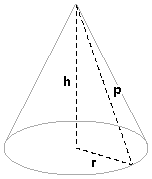The cone's surface is made of two parts: the base and the side.Our formula is the sum of the two areas:

Acone = Abase + Aside

### The Area of the Base

The base is a disk of radius r. Its area is pi × r2. Our area formula is now

Acone = pi × r2 + Aside

### The Area of the Side

Here's where we use our insight. We can transform the cone's side into a circular disk minus a sector by cutting along p and flattening: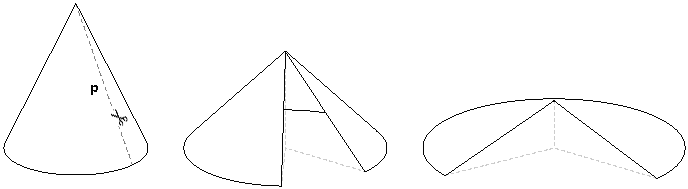We end up with a disk minus a sector.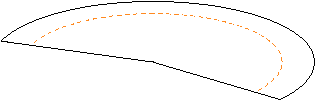Note that we're viewing it in perspective. From above it looks like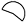.

(If flattening the cone's side is hard for you to imagine, try this. Cut the side along p as before. Turn the cone on its side so that the apex lies on the ground. Pin down one of the edges you created by cutting along p. Unroll the cone around its apex. The result is the same as if we'd flattened it.)

What is the area of this shape? We know that the area of a disk with radius p is pi × p2. But that doesn't take into account the missing sector. What is its area?

Note the dotted line in the figure above. Consider it as a measure of how much of the disk is actually there (as opposed to missing). If it's 1, then we have a complete disk; if it's 1/2, then we have half a disk. (Precisely, it's the ratio of the area of the disk-minus-sector to the full disk.) To find the area of the disk-minus-sector, take the area of the full disk [pi × p2] and multiply it by this ratio. The problem is now to figure out what the ratio is.

From the construction above, the circumference of the base of the cone and curved side of the disk-minus-sector are the same length. The corresponding parts are shown below in red.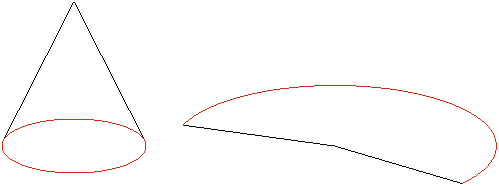Since we know the circumference of the base of the cone [2 × pi × r], the curved part of the disk is in ratio (2 × pi× r) / (2 × pi × p) = r/p to the entire disk. Multiplying this ratio by the area of the full disk gives (r/p) × pi × p2 = pi × r × p. This is the area of the side.

p can be determined by the Pythagorean theorem [r2 + h2 = p2]. Taking the square root of both sides gives p = (r2 + h2)1/2.

## The Formula

Adding it all up gives

Acone = pi × r2 + pi × r × p

Factoring out terms:

Acone = pi × r × (r + p)

Substituting for p gives the solution:

Acone = pi × r × (r + (r2 + h2)1/2)

## Checking for Correctness

Let's do a quick sanity check on the formula. We would expect that as h increases, so does the surface area. Does it? As h increases, so does p, so the surface does increase. Good.

Now, what happens as h decreases to zero? The cone gets flatter and flatter, with the tip approaching the base. So the surface area should approach two times the area of the base. If we plug in zero for h, we get pi × r × (r + r) = 2 × pi × r2. Looks like we have a winner.

## Epilogue

Now that all that's over, I have to confess that I wrote the page not only because I like this derivation but because I can never remember the formula.

Plane geometry formulas

1. The circumference of a circle of radius r is 2 × pi × r

2. The area of a disk of radius is r is pi × r2

3. Given a right triangle with sides a, b, and hypotenuse c, a2 + b2 = c2 [The Pythagorean theorem]

Last updated 5 September 2004
http://www.rdrop.com/~half/Creations/Puzzles/ConeArea/index.html
All contents ©1999-2003 Mark L. Irons